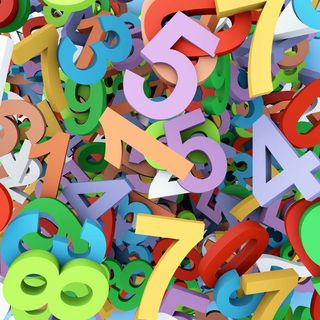# Real Numbers: Properties and DefinitionReal numbers are, in fact, pretty much any number that you can think of. This can include whole numbers or integers, fractions, rational numbers and irrational numbers. Real numbers can be positive or negative, and include the number zero. They are called real numbers because they are not imaginary, which is a different system of numbers.

Imaginary numbers are numbers that cannot be quantified, like the square root of -1. The number, denoted as i, can be used for equations and formulas, but is not a real number that can be used in basic arithmetic. You cannot add or subject imaginary numbers. Another example of an imaginary number is infinity.

## Any point on a line

One identifying characteristic of real numbers is that they can be represented over a number line. Think of a horizontal line. The center point, or the origin, is zero. To the right are all positive numbers, and to the left are the negative points. Any point on the line would be considered a real number. You can find a rational number on this line, like ¾ or 72.3, and you’ll also find an irrational number like pi. Because they lie on a line, their sizes can be compared. One can be greater or less than another, they can be ordered, and you can use them in addition, subtraction, multiplication and division.

Therefore, all of these rational and irrational numbers, including fractions, are considered real numbers. Real numbers that include decimal points are known as floating point numbers because the decimal floats within the numbers. Integers or whole numbers cannot be floating point numbers.